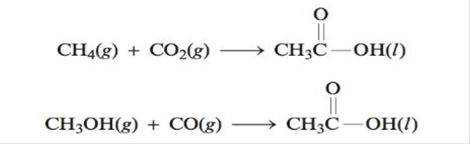Chapter 17, Problem 63E

Chapter
Section
Textbook Problem

# Using data from Appendix 4, calculate ∆H°, ∆S° and ∆G° for the following reactions that produce acetic acid:Which reaction would you choose as a commercial method for producing acetic acid (CH3CO2H) at standard conditions? What temperature conditions would you choose for the reaction? Assume ∆H° and ∆S° do not depend on temperature.

Interpretation Introduction

Interpretation: The values of ΔHο,ΔSο and ΔGο for the given reactions are to be calculated. The reaction that involves a commercial method for producing acetic acid at standard condition is to be stated. The temperature conditions are to be stated. The independency of ΔHο and ΔSο on the temperature is to be assumed.

Concept introduction: The term ΔGο is a thermodynamic function. The superscript on this function represents its standard form. The term Δ represents the change. This function is known as the standard Gibb’s free energy change. It correlates the enthalpy and entropy of the system in a mathematical formula.

Explanation

Explanation

Given

The reactions are given as,

2CH4(g)+CO2(g)CH3COOH(l)CH3OH(g)+CO(g)CH3COOH(l)

The given values of ΔHfο,ΔSfο and ΔGfο are as follows,

SubstanceΔHfο(kJ/mol)ΔSfο(J/Kmol)ΔGfο(kJ/mol)CH3COOH(l)484160389CH4(g)7518651CH3OH(g)201240163CO2(g)393.5214394CO(g)110.5198137

The calculation of standard enthalpy change for both the reactions is given below.

The value of standard enthalpy change ΔHο of the given reaction is calculated by the formula,

ΔHο=npΔHfο(products)nrΔHfο(reactants)

Where,

• ΔHfο(reactants) are the standard free energy of formation for the reactants.
• ΔHfο(products) are the standard free energy of formation for the products.
• np is the number of products molecule.
• nr is the number of reactants molecule.
• is the symbol of summation.

For the first reaction, the representation in the above form is written as,

ΔHο=npΔHfο(products)nrΔHfο(reactants)ΔHο=ΔHfοCH3COOH(l)ΔHfοCH4(g)ΔHfοCO2(g)

Substitute the values of standard enthalpy of formations in the above equation.

ΔHο=ΔHfοCH3COOH(l)ΔHfοCH4(g)ΔHfοCO2(g)ΔHο=1mol(484kJ/mol)1mol(75kJ/mol)1mol×(393kJ/mol)ΔHο=(484kJ)+(75kJ)+(393kJ)ΔHο=16kJ_

For the second reaction, the representation in the above form is written as,

ΔHο=npΔHfο(products)nrΔHfο(reactants)ΔHο=ΔHfοCH3COOH(l)ΔHfοCH3OH(g)ΔHfοCO(g)

Substitute the values of standard enthalpy of formations in the above equation.

ΔHο=ΔHfοCH3COOH(l)ΔHfοCH3OH(g)ΔHfοCO(g)ΔHο=1mol(484kJ/mol)1mol(201kJ/mol)1mol×(110.5kJ/mol)ΔHο=(484kJ)+(201kJ)+(110.5kJ)ΔHο=173kJ_

The calculation of standard entropy change for both the reactions is given below.

The value of standard entropy change ΔSο of the given reaction is calculated by the formula,

ΔSο=npΔSfο(products)nrΔSfο(reactants)

Where,

• ΔSfο(reactants) are the standard free energy of formation for the reactants.
• ΔSfο(products) are the standard free energy of formation for the products.
• np is the number of products molecule.
• nr is the number of reactants molecule.
• is the symbol of summation.

For the first reaction, the representation in the above form is written as,

ΔSο=npΔSfο(products)nrΔSfο(reactants)ΔSο=ΔSfοCH3COOH(l)ΔSfοCH4(g)ΔSfοCO2(g)

Substitute the values of standard entropy of formations in the above equation.

ΔSο=ΔSfοCH3COOH(l)ΔSfοCH4(g)ΔSfοCO2(g)ΔSο=1mol(160J/Kmol)1mol(186J/Kmol)1mol×(214J/Kmol)ΔSο=(160J/Kmol)(186J/Kmol)(214J/molK)ΔSο=240J/K_

For the second reaction, the representation in the above form is written as,

ΔSο=npΔSfο(products)nrΔSfο(reactants)ΔSο=ΔSfοCH3COOH(l)ΔSfοCH3OH(g)ΔSfοCO(g)

Substitute the values of standard entropy of formations in the above equation

### Still sussing out bartleby?

Check out a sample textbook solution.

See a sample solution

#### The Solution to Your Study Problems

Bartleby provides explanations to thousands of textbook problems written by our experts, many with advanced degrees!

Get Started